Kindergarten PDF Math Worksheets

No prep PDF worksheets designed to impact Kindergarteners into becoming life long math learners!

Kindergarten is the ideal time to build on preschool success and prepare for complex math. These appealing activity workbooks review basic math topics such as ordering numbers, counting, and number lines. Then develop confidence in addition and subtraction with inviting daily practice worksheets. Build the foundation for first grade success with the dynamic tools to make your own workbooks!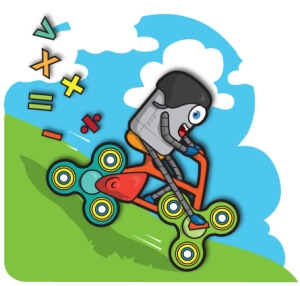Monthly Math Challenge Workbooks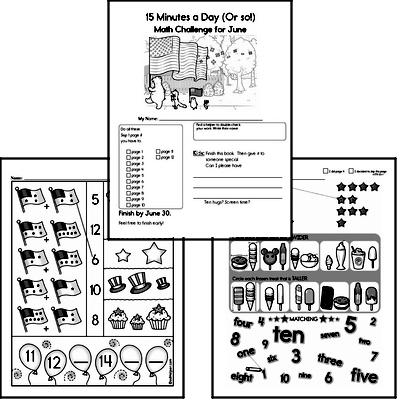Kindergarten Math Challenge Book

Kindergarten Math Worksheets Activity Workbook - No Prep and Not Boring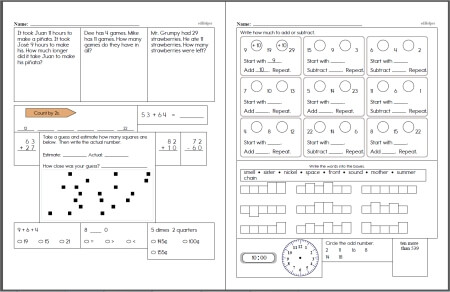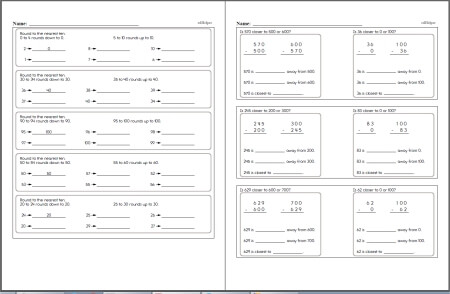Kindergarten Math Book New Kindergarten Math Workbook Created Use it each week for daily practice in your classroom.

Kindergarten Geometry

Order Numbers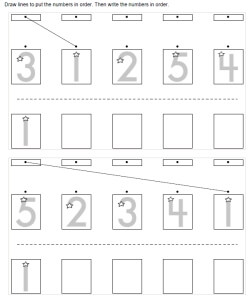Ordering five numbers (numbers 1 to 5) Ordering five numbers (numbers can be from 1 to 9)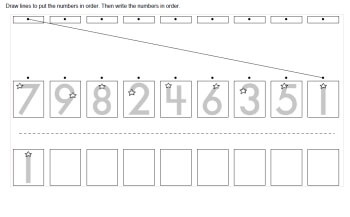Ordering nine numbers (numbers 1 to 9)

Counting

Number Lines

Calendar Skills:  Practice Writing a Calendar Each Month

Comparing Numbers

Subtraction and Introduction to Subtraction

Color by Number

Color by Subtraction

Skip Counting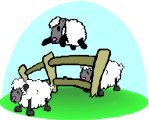Skip Counting Reading Comprehensions (grades 1-2 reading level)

Introduction to Math
 Mazes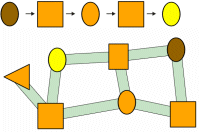Number Mazes and Shapes Mazes

 Matching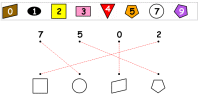Matching Shapes, Numbers, and Colors

 Combined Shapes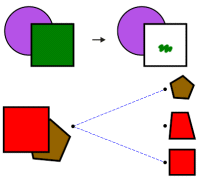Combined Shapes: Coloring and Matching

 Ordering Animals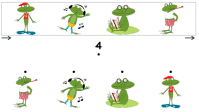Ordering Animals

 Ordering Shapes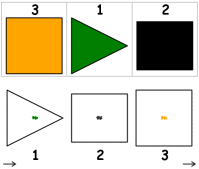Ordering Shapes

 Counting - Part 1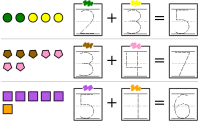Math Counting (part 1)

 Counting - Part 2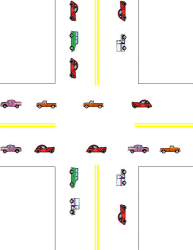Math Counting (part 2)

 Grids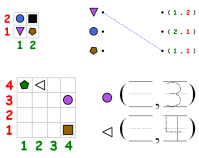Grids: Shapes, Positions, Writing Numbers

 Greater and Less Than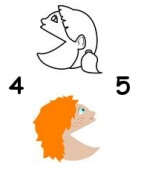Greater and Less Than

Hundreds Chart

Hundreds Chart Pieces Puzzle

Have a suggestion or would like to leave feedback?